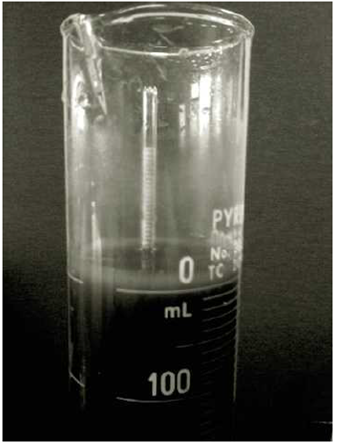Chapter 2, Problem 2.14PPrinciples of Geotechnical Enginee...

9th Edition
Braja M. Das + 1 other
ISBN: 9781305970939

Solutions

Chapter
SectionPrinciples of Geotechnical Enginee...

9th Edition
Braja M. Das + 1 other
ISBN: 9781305970939
Textbook Problem

A hydrometer test has the following result: Gs = 2.65, temperature of water = 26° C, and L = 10.4 cm at 45 minutes after the start of sedimentation (see Figure 2.25). What is the diameter D of the smallest-size particles that have settled beyond the zone of measurement at that time (that is, t = 45 min)?Figure 2.25 ASTM 152H type of hydrometer placed inside the sedimentation cylinder (Courtesy of Khaled Sobhan, Florida Atlantic University, Boca Raton, Florida)

To determine

Calculate the diameter of the smallest-size particles that have settled beyond the zone of measurement at 45 min time duration.

Explanation

Given information:

The specific gravity (Gs) of the particle is 2.65.

The temperature of water is 26°C.

The depth measured from the surface of the water to the center of gravity of the hydrometer bulb (L) is 10.4cm.

The time duration (t) is 45min.

Calculation:

Consider K is a constant and a function of specific gravity and viscosity of water.

Calculate the value of K as shown below.

Refer to Table 2.9 “Values of K” in the Text book.

For the corresponding value of specific gravity 2.65 and temperature 26°C, the K value is 0.01272.

Calculate the diameter D of the smallest-size particle using the relation

Still sussing out bartleby?

Check out a sample textbook solution.

See a sample solution

The Solution to Your Study Problems

Bartleby provides explanations to thousands of textbook problems written by our experts, many with advanced degrees!

Get Started

Find more solutions based on key concepts